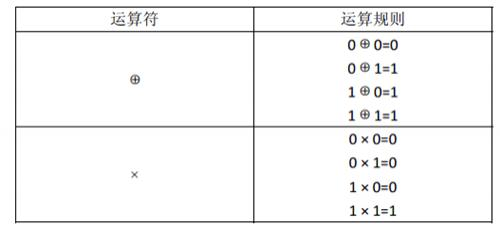# 【数据结构】P1310 表达式的值

【题目链接】

https://www.luogu.org/problem/P1310

### 题目描述1. 先计算括号内的，再计算括号外的。

2. “× ”运算优先于“⊕”运算，即计算表达式时，先计算× 运算，再计算⊕运算。例如：计算表达式A⊕B × C时，先计算 B × C，其结果再与 A 做⊕运算。

### 输入输出样例

4
+(*)


３


### 说明/提示

【输入输出样例说明】

【题解】

1、转变为后缀表达式的形式，然后，如果是操作数要添加一个数字，记住第一个位置要多添加一个‘.’

2、然后进行推导，把四种情况的转移状态写清楚。

## 第一步：中缀转后缀

1）如果遇到操作数，我们就直接将其输出。

2）如果遇到操作符，则我们将其放入到栈中，遇到左括号时我们也将其放入栈中。

3）如果遇到一个右括号，则将栈元素弹出，将弹出的操作符输出直到遇到左括号为止。注意，左括号只弹出并不输出。

4）如果遇到任何其他的操作符，如（“+”， “*”，“（”）等，从栈中弹出元素直到遇到发现更低优先级的元素(或者栈为空)为止。弹出完这些元素后，才将遇到的操作符压入到栈中。有一点需要注意，只有在遇到" ) "的情况下我们才弹出" ( "，其他情况我们都不会弹出" ( "。

5）如果我们读到了输入的末尾，则将栈中所有元素依次弹出。

## 第二步：DP

#### 如果当前是"*"：

（注意两式顺序不能颠倒，因为1式中需要用到f[now]

（两式的顺序同样不能颠倒）

#### 其中，

【代码】1 #include<bits/stdc++.h>
2 using namespace std;
3 const int mod = 10007 ;
4 const int N = 1e5+10;
5
6 int f[N] ;
7
8 int priority( char s ){
9     switch ( s ){
10         case '+' : return 1 ;
11         case '*' : return 2 ;
12         case '(' :
13         case ')' : return -1;
14     }
15 }
16
17 void toSuffixString( string &s ){
18     int len = s.length();
19
20     string res = "." ;
21     stack < int > Op ;
22
23     for(int i=0;i<len ;i++){
24         /*if( s[i] == '.') {
25             res += ".";
26         }else */
27         if( s[i] == '(' || s[i] == '*' ){
28             Op.push(s[i]);
29         }else if( s[i] == ')' ){
30             while( !Op.empty() && Op.top() != '(' ){
31                 res += Op.top();
32                 Op.pop();
33             }
34             //出来的时候，栈顶为(.
35             Op.pop();
36         }else{
37             while( !Op.empty() && priority(Op.top()) >= priority(s[i]) ){
38                 res += Op.top();
39                 Op.pop();
40             }
41             //出来的时候，栈里面的运算符优先级低于当前位置.
42             Op.push( s[i] ) ;
43         }
44         if( !(s[i] == '(' || s[i] == ')') ){
45             res += ".";
46         }
47     }
48     while( !Op.empty() ){
49         res += Op.top();
50         Op.pop();
51     }
52     //cout << res << endl ;
53     s = res ;
54 }
55
56 void Calc( string s ){
57     //cout << s << endl ;
58     int j = 0 ;
59     int len = s.length();
60     for(int i=0 ; i < len ; i++ ){
61         if( s[i] == '.' ){
62             j ++ ;
63             f[j] = f[j] = 1 ;
64         }else if( s[i] == '*' ){
65             j -- ;
66             f[j] =(f[j] * f[j+1] +
67                       f[j] * f[j+1] +
68                       f[j] * f[j+1] ) % mod ;
69
70             f[j] = (f[j] * f[j+1]) % mod ;
71
72         }else if( s[i] == '+' ){
73             j -- ;
74             f[j] =(f[j] * f[j+1] +
75                       f[j] * f[j+1] +
76                       f[j] * f[j+1] ) % mod ;
77
78             f[j] =(f[j] * f[j+1] ) %mod ;
79         }
80     }
81     cout << f << endl;
82 }
83
84 int main(){
85     ios_base :: sync_with_stdio( false );
86     cin.tie(NULL) , cout.tie(NULL) ;
87     int n ;
88     string str ;
89     cin >> n >> str ;
90     toSuffixString(str);
91     Calc(str);
92     return 0;
93 }
View Code

posted @ 2019-08-20 02:15 Osea 阅读(...) 评论(...) 编辑 收藏